# nth term of a GP

nth term of a GP

Hey kids! Meet Sam's family.

Here is his family tree.Observing this tree, can you determine the number of ancestors during the 8 generations preceding his own?

You will have to use the concept of geometric sequence to answer this.

Don't worry! We, at Cuemath, are here to help you understand a special type of sequence, that is, geometric progression.

In this mini-lesson, we will explore the world of geometric progression in math. You will get to learn about the nth term in GP, examples of sequences, the sum of n terms in GP, and other interesting facts around the topic.

## Lesson Plan

 1 What Is Meant By Geometric Progression? 2 Important Notes 3 Solved Examples on Geometric Progression 4 Thinking Out of the Box! 5 Interactive Questions on Geometric Progression

## What Is Meant by Geometric Progression?

A geometric sequence is a sequence where every term bears a constant ratio to its preceding term.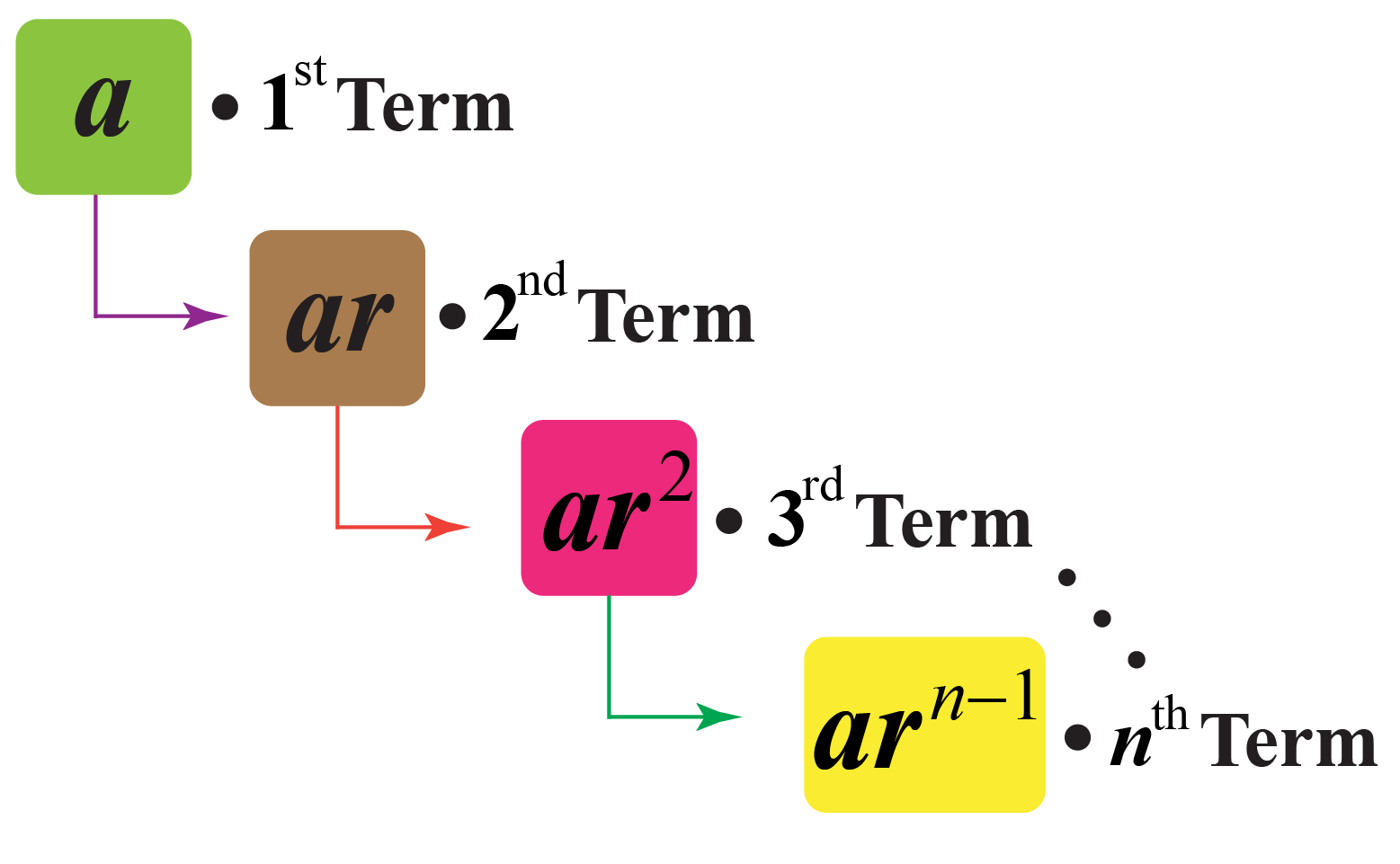### Example

Consider an example of geometric sequence: 1, 4, 16, 64, ...

Observe that $$\dfrac{4}{1}=\dfrac{16}{4}=\dfrac{64}{16}=4$$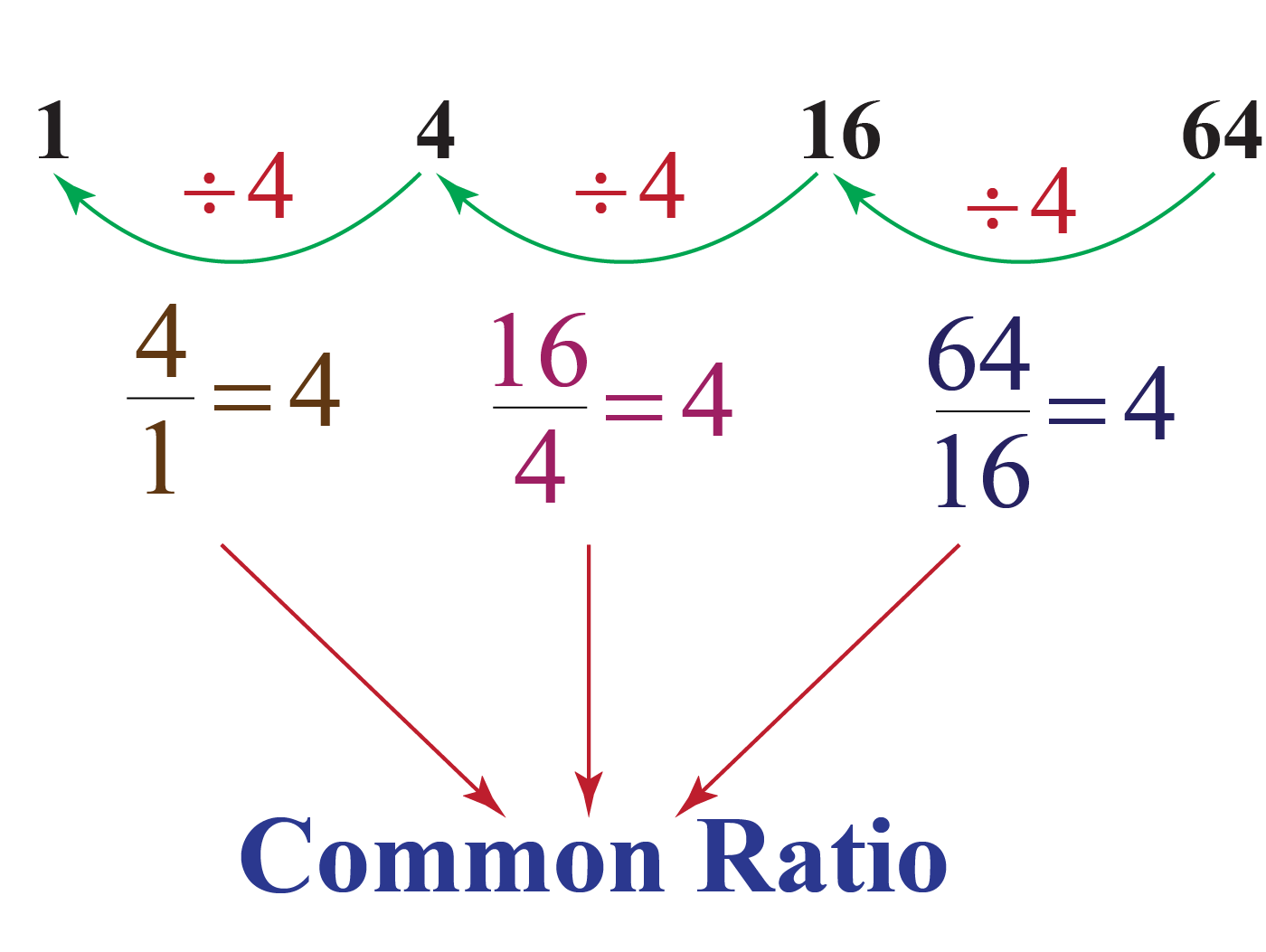Here, 4 is a common ratio.

## How To Determine the nth Term of GP?

Let the first term of the sequence be $$a$$ and the common ratio be $$r$$.

Then, $$n^{\text{th}}$$ term of the sequence is given by:

 \begin{align}a_{n}=ar^{n-1}\end{align}

Use the below simulation to calculate the nth term of some geometric progressions.

Input the values of $$n$$ for the number of terms you want to calculate.

### Geometric Progression: Sum

Consider a geometric progression whose first term is $$a$$ and the common ratio is $$r$$.

The sum of the first $$n$$ terms of a geometric progression is:

 $$S_n=\dfrac{a(1-r^n)}{1-r}$$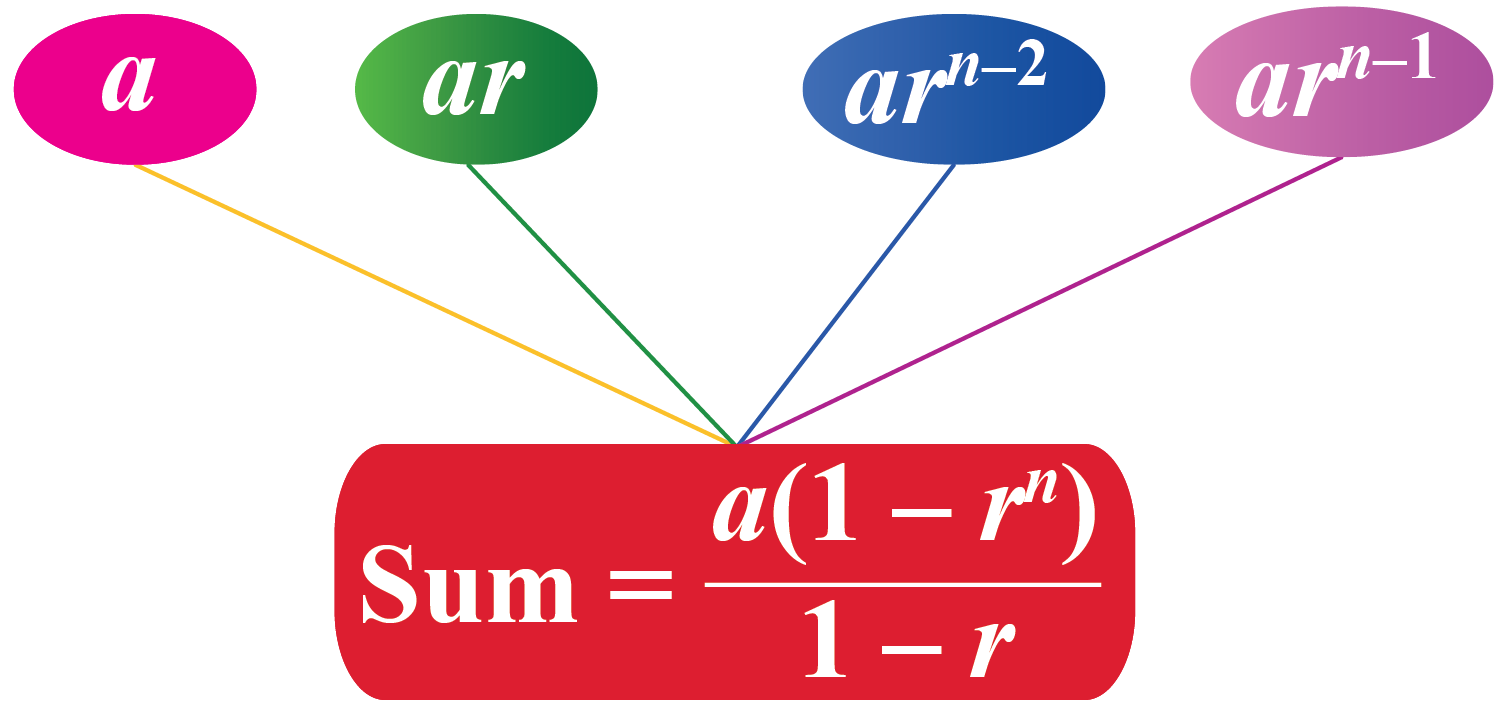### Example

Can you calculate the nth term of the geometric progression if the first two terms are 10 and 20?

First, you need to calculate the common ratio $$r$$ of the geometric series by dividing the second term by the first term.

\begin{aligned}r&=\frac{20}{10}\\&=2\end{aligned}

Then substitute the values of the first term $$a$$ and the common ratio $$r$$ into the formula of the nth term of the geometric progression $$a_{n}=ar^{n-1}$$.

\begin{aligned}a_{n}&=ar^{n-1}\\&=10(2)^{n-1}\\&=10\frac{2^{n}}{2}\\&=5(2)^{n}\end{aligned}

## Solved Examples

 Example 1

Look at the pattern shown below.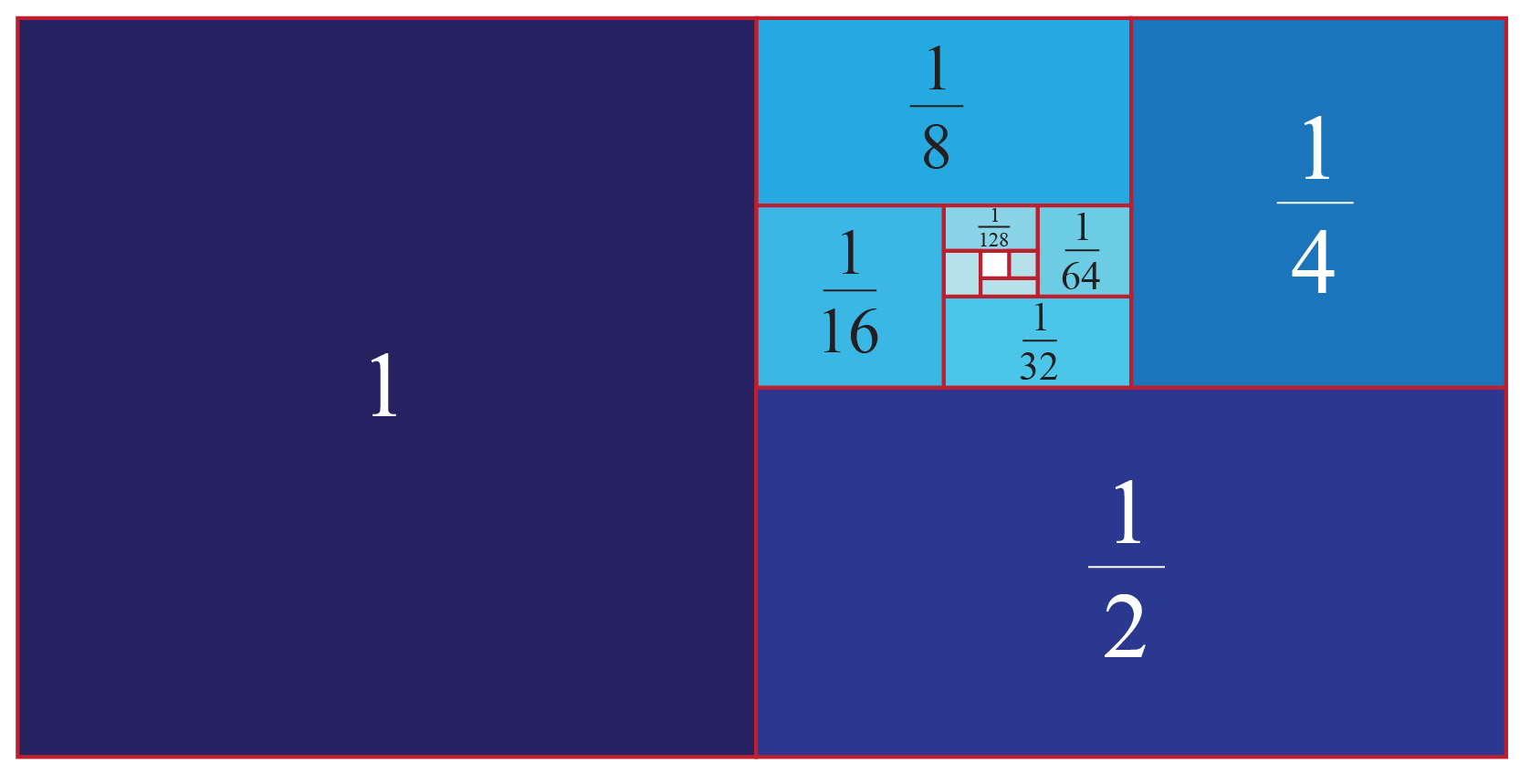Observe that each square is half of the size of the square next to it.

Which sequence does this pattern represent?

### Solution

Let's write the sequence represented in the figure.

$1, \dfrac{1}{2}, \dfrac{1}{4}, \dfrac{1}{8}, \dfrac{1}{16},...$

Every successive term is obtained by dividing its preceding term by 2

The sequence exhibits a common ratio of $$\dfrac{1}{2}$$

 $$\therefore$$ The pattern represents the geometric progression.
 Example 2

Hailey's teacher asks her to find the $$10^{\text{th}}$$ term of the sequence: 1, 3, 9, 27, ...Can you help her?

Solution

Observe that $$\dfrac{3}{1}=\dfrac{9}{3}=\dfrac{27}{9}=3$$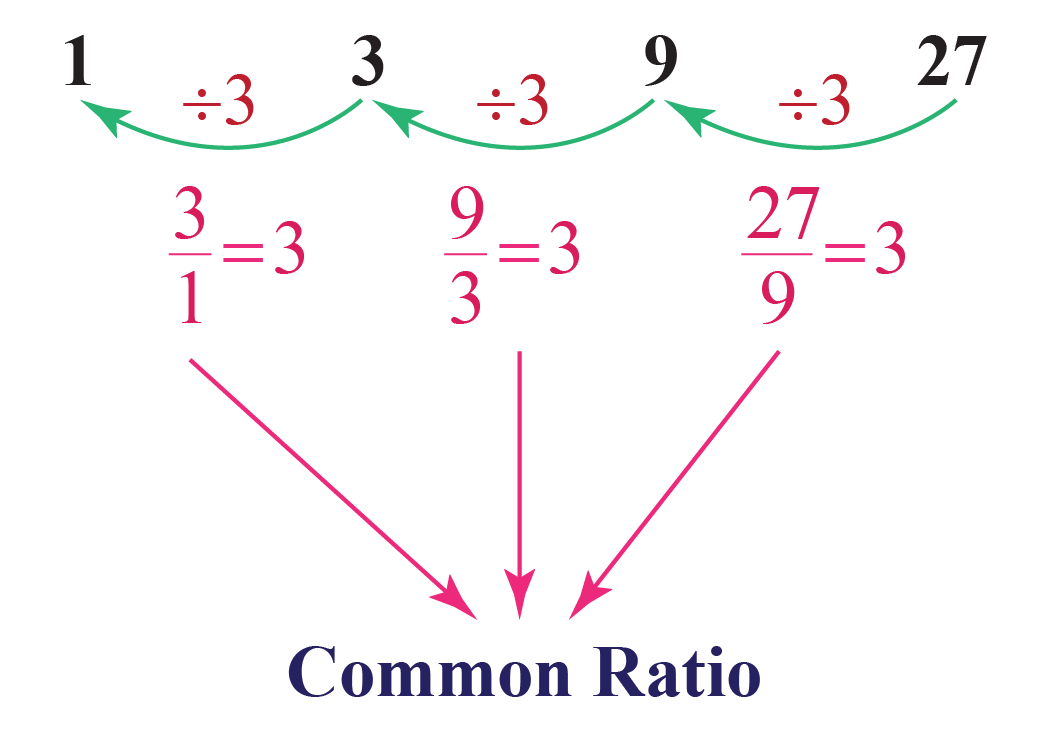Here, $$r=3$$ is a common ratio.

So, the given sequence represents the geometric progression.

The 10th term of the sequence will be given by $$ar^{9}$$.

\begin{align}ar^{9}&=1\times 3^9\\&=3^9\end{align}

 $$\therefore$$ The 10th term of the sequence is $$3^9$$
 Example 3

If the nth term of a GP is 128 and both the first term $$a$$ and the common ratio $$r$$ are 2

Can you calculate the total number of terms in the GP?

Solution

Given,

The nth term of a GP is $$a_n=128$$

The first term of the GP is $$a=2$$

The common ratio of the GP is $$r=2$$

Now use the condition if the first and nth term of a GP are a and b respectively then, $$b=a\cdot r^{n-1}$$, to calculate the total number of terms.

\begin{align}a_n&=a\cdot r^{n-1}\\128&=2\cdot 2^{n-1}\\64&=2^{n-1}\\2^{6}&=2^{n-1}\\6&=n-1\\n&=7\end{align}

 $$\therefore$$ There are 7 terms in the GP.Think Tank
 1. There are 25 trees at equal distances of 5 feet in a line with a well, the distance of the well from the nearest tree being 10 feet. A gardener waters all the trees separately starting from the well and he returns to the well after watering each tree. Find the total distance the gardener will cover.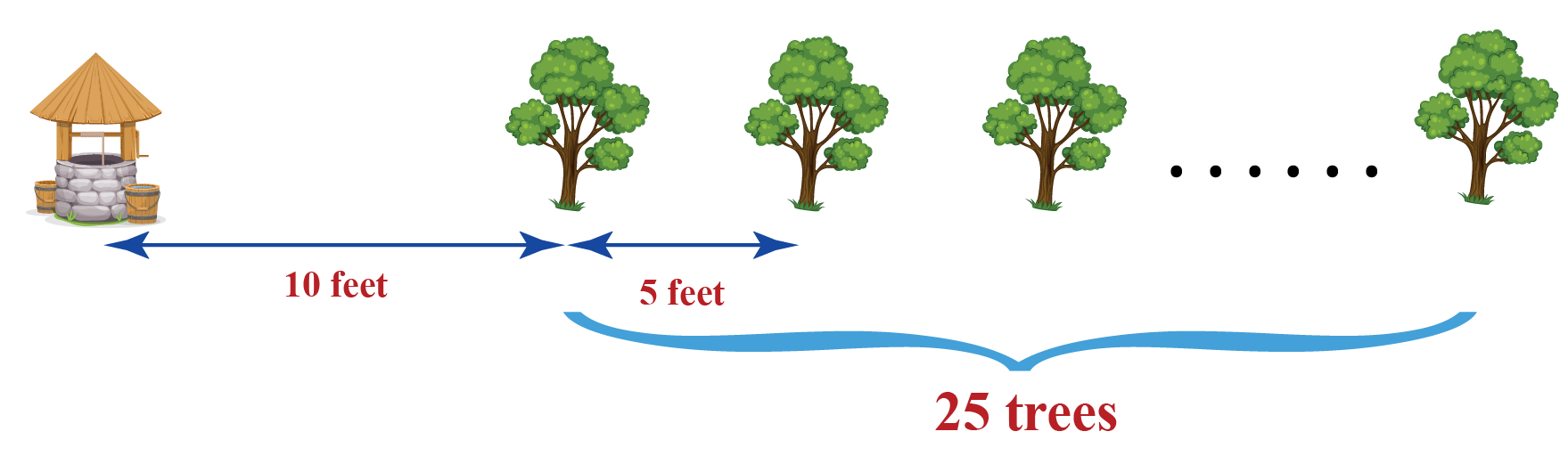## Interactive Questions

Here are a few activities for you to practice.

## Let's Summarize

This mini-lesson targeted the fascinating concept of the nth term of GP. The math journey around nth term in GP starts with what a student already knows, and goes on to creatively crafting a fresh concept in the young minds. Done in a way that is not only relatable and easy to grasp but will also stay with them forever. Here lies the magic with Cuemath.

At Cuemath, our team of math experts is dedicated to making learning fun for our favorite readers, the students!

Through an interactive and engaging learning-teaching-learning approach, the teachers explore all angles of a topic.

Be it worksheets, online classes, doubt sessions, or any other form of relation, it’s the logical thinking and smart learning approach that we, at Cuemath, believe in.

## FAQs

### 1. What is the full form of GP?

The full form of GP is, "Geometric Progression".

### 2. What does R equal in geometric progression?

In geometric progression, R is the common ratio of the two consecutive terms.

### 3. How do you identify a geometric sequence?

Calculate the ratio of the successive terms of the sequence with the corresponding preceding terms. If all the ratios are equal then the sequence is a geometric sequence.

### 4. What does the nth term mean?

The nth term represents the general term of the sequence such that $$n=1,2,3,...$$ gives the first term, the second term, the third term,... of the sequence.

### 5. What is the formula for finding the nth term?

The nth term of a geometric sequence with first term $$a$$ and the common ratio $$r$$ is given by $$a_{n}=ar^{n-1}$$.

### 6. How do you find the nth term of a geometric progression with two terms?

First, calculate the common ratio $$r$$ by dividing the second term by the first term. Then use the first term $$a$$ and the common ratio $$r$$ to calculate the nth term by using the formula $$a_{n}=ar^{n-1}$$.

### 7. What is the difference between arithmetic and geometric progression?

The arithmetic progression has a common difference between each consecutive term. While geometric progression has a common ratio between each consecutive term.

### 8. How do you solve geometric progression?

To solve the geometric progression first calculate the common ratio $$r$$, then use the first term and the common ratio to calculate the desired terms.

### 9. What is the formula of geometric progression?

The formula of geometric progression is $$a_{n}=ar^{n-1}$$, where $$a$$ ane $$r$$ are the first term and the common ratio respectively.

### 10. What is the formula for the sum of n terms in GP?

The sum of geometric progression with first term $$a$$ and the common ratio $$r$$ is given by $$S_n=\dfrac{a(1-r^n)}{1-r}$$.

More Important Topics
More Important Topics